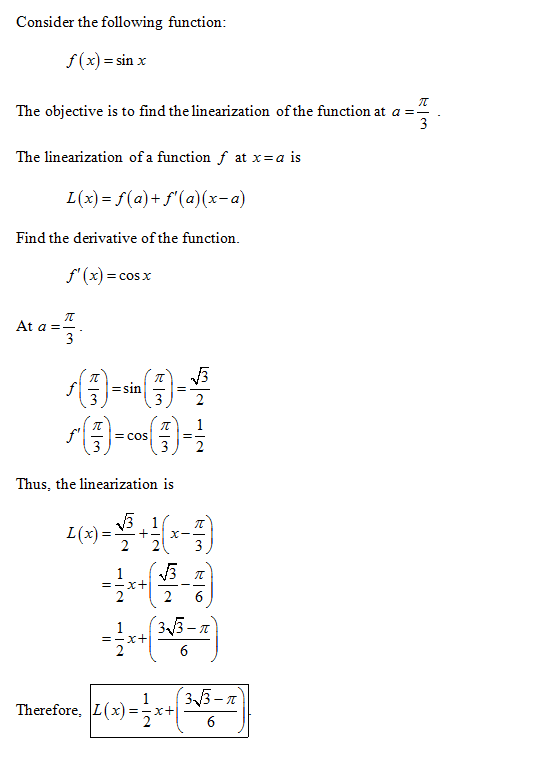# Find the linearization l(x) of the function at a. f(x) = sin(x). a = π 3

Find the linearization L(x) of the function at a. f(x)= sin(x),
a= pi/3

Consider the following function The objective is to find the linearization ofthe function at a =- The linearization of a function f at x=a is し(x)[email protected]'(a)(x-a) Find the derivative ofthe function (x)=cos x At a=- 32 Thus, the linearization is Therefore, IL(x)=-x+]